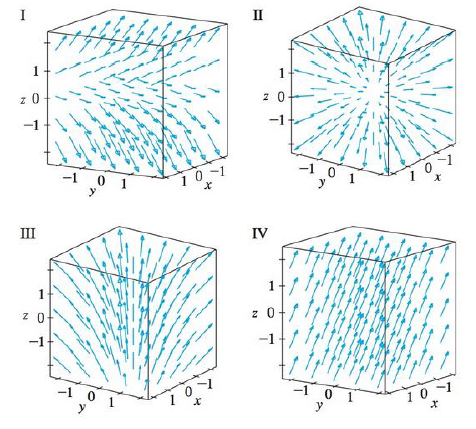Chapter 16.1, Problem 15E

Chapter
Section
Textbook Problem

Match the vector fields F on ℝ3 with the plots labeled I-IV. Give reasons for your choices.15. F(x, y, z) = i + 2 j + 3 kTo determine

To match: The vector field F(x,y,z)=i+2j+3k with the plots labeled as I-IV.

Explanation

Given data:

F(x,y,z)=i+2j+3k=1,2,3

Formula used:

Consider a three-dimensional vector F=x,y,z .

Write the expression for length of the three dimensional vector.

|F(x,y,z)|=x2+y2+z2 (1)

Find the length of F(x,y,z) using equation (1)

Still sussing out bartleby?

Check out a sample textbook solution.

See a sample solution

The Solution to Your Study Problems

Bartleby provides explanations to thousands of textbook problems written by our experts, many with advanced degrees!

Get Started

Find more solutions based on key concepts